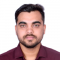## How to specify different colors for different bars in a Python matplotlib histogram?Rishikesh Kumar Rishi

Updated on 01-Feb-2022 11:44:04

To specify different colors for different bars in a matplotlib histogram, we can take the following steps −StepsSet the figure size and adjust the padding between and around the subplots.Create a figure and a set of subplots.Plot a histogram with random data with 100 sample data.Iterate in the range of ... Read More

## How do I fill a region with only hatch (no background colour) in matplotlib 2.0?Rishikesh Kumar Rishi

Updated on 01-Feb-2022 11:40:47

To fill a region with only hatch (no background color) in matplotlib, we can take the following steps −StepsSet the figure size and adjust the padding between and around the subplots.Initialize a variable n to store the number of sample data.Create a figure and a set of subplots.Plot the x ... Read More

## How to set a line color to orange, and specify line markers in Matplotlib?Rishikesh Kumar Rishi

Updated on 01-Feb-2022 11:37:53

To set a line color to orange, and specify line markers in matplotlib, we can take the following steps −StepsSet the figure size and adjust the padding between and around the subplots.Create x and y data points using numpy.Plot the x and y data points with the attributes color='orange' and ... Read More

## How to deal with NaN values while plotting a boxplot using Python Matplotlib?Rishikesh Kumar Rishi

Updated on 01-Feb-2022 11:35:26

To deal with NaN value while plotting a boxplot using Python, we can take the following steps −StepsSet the figure size and adjust the padding between and around the subplots.Initialize a variable N for data samples and for range.Next create the random spread, center's data, flier high and low, get ... Read More

## How to plot a smooth line with matplotlib?Rishikesh Kumar Rishi

Updated on 01-Feb-2022 11:32:09

To plot a smooth line with matplotlib, we can take the following steps −StepsSet the figure size and adjust the padding between and around the subplots.Create a list of data points, x and y.Plot the x and y data points.Create x_new and bspline data points for smooth line.Get y_new data ... Read More

## Representing voxels with matplotlibRishikesh Kumar Rishi

Updated on 01-Feb-2022 11:29:01

In 3D computer graphics, a voxel represents a value on a regular grid in three-dimensional space. We can say a voxel is a 3D equivalent of a pixel that is used in 2D. A pixel is a square inside of a 2D image with a position in a 2D grid ... Read More

## How to get a Gantt plot using matplotlib?Rishikesh Kumar Rishi

Updated on 01-Feb-2022 11:25:48

Gantt charts are widely used in project planning to show the project schedule. It's a type of bar chart that lists the tasks on the vertical axis and the time intervals on the horizontal axis. The width of the horizontal bars in the graph shows the duration of each activity.To ... Read More

## How to avoid line color repetition in matplotlib.pyplot?Rishikesh Kumar Rishi

Updated on 01-Feb-2022 11:23:09

To avoid line color repetition in matplotlib.pyplot we can take the following steps −StepsSet the figure size and adjust the padding between and around the subplots.Create x and y data points using numpy.Plot the x and y data points using plot() method.In the plot() method, use a unique hexadecimal value ... Read More

## How to visualize values on logarithmic scale on matplotalib?Rishikesh Kumar Rishi

Updated on 01-Feb-2022 11:20:03

To visualize values on logarithmic scale on matplotlib, we can use yscale('log').StepsImport matplotlib nd numpy.Set the figure size and adjust the padding between and around the subplots.Create x and y data points using numpy.Use yscale('log') to visualize the values on a logarithmic scale.Plot x and y data points using plot ... Read More

## How to change xticks font size in a matplotlib plot?Rishikesh Kumar Rishi

Updated on 01-Feb-2022 11:17:06

To change the font size of xticks in a matplotlib plot, we can use the fontsize parameter.StepsImport matplotlib and numpy.Set the figure size and adjust the padding between and around the subplots.Create x and y data points using numpy.Plot the x and y data points using plot() method.Set the font ... Read More